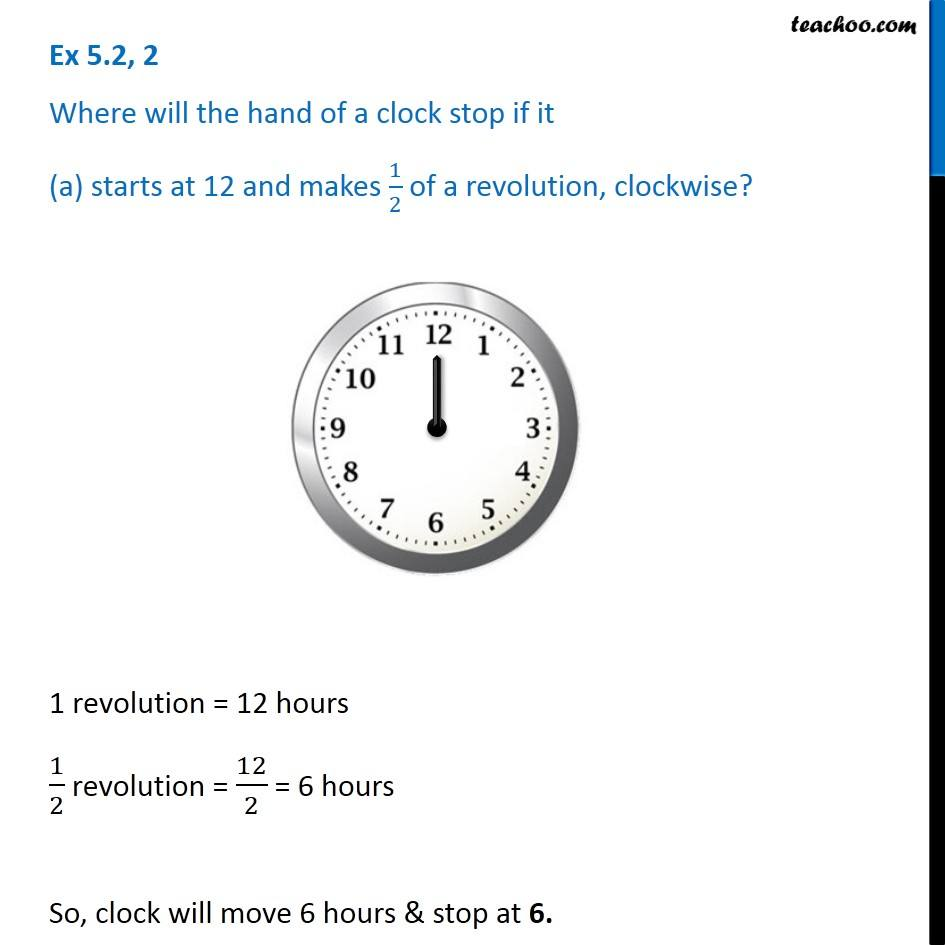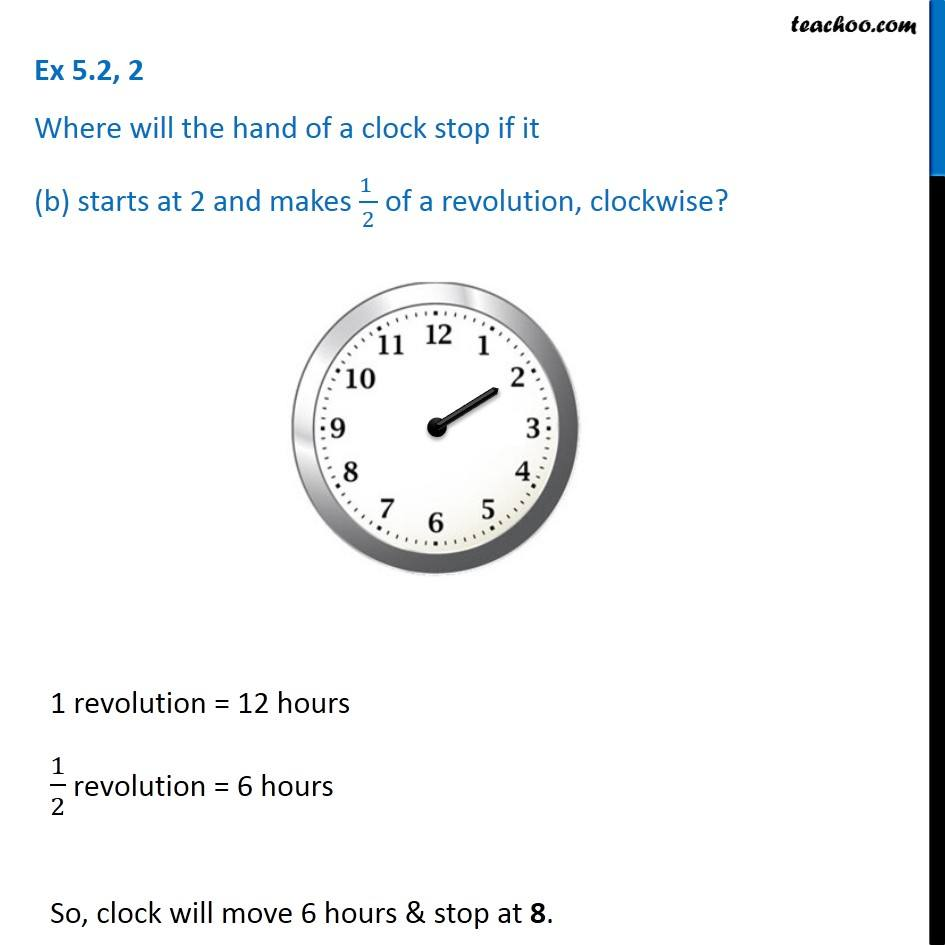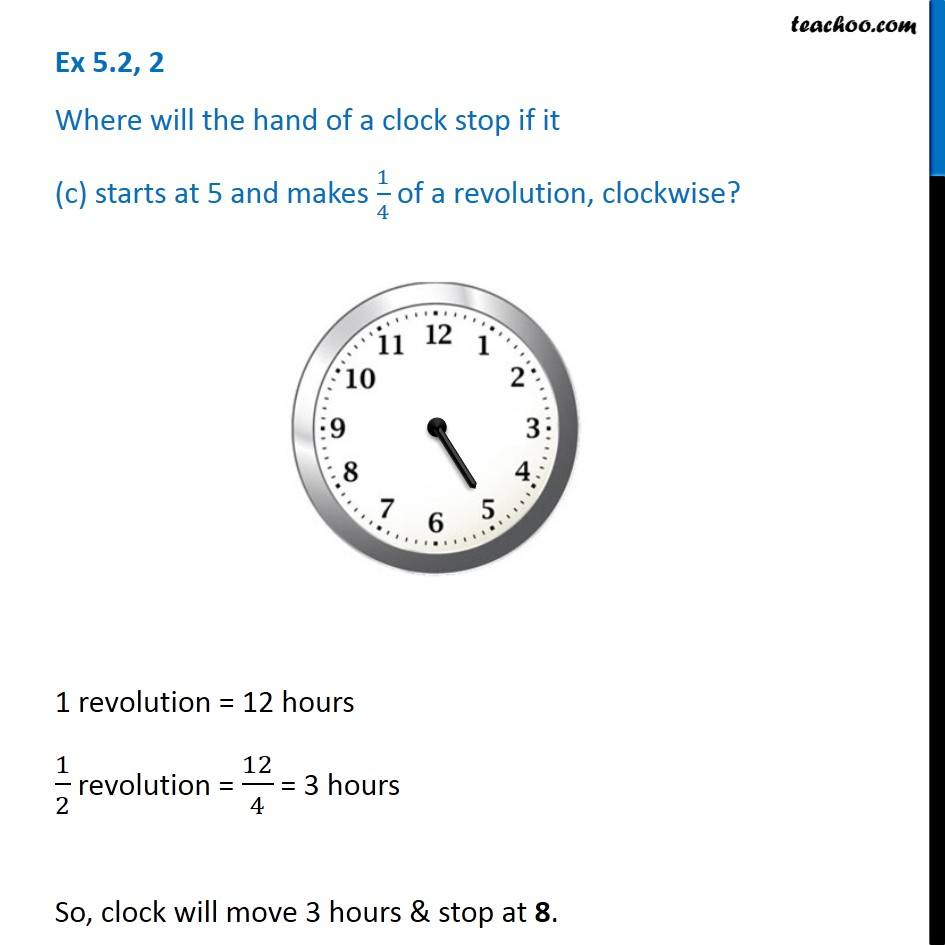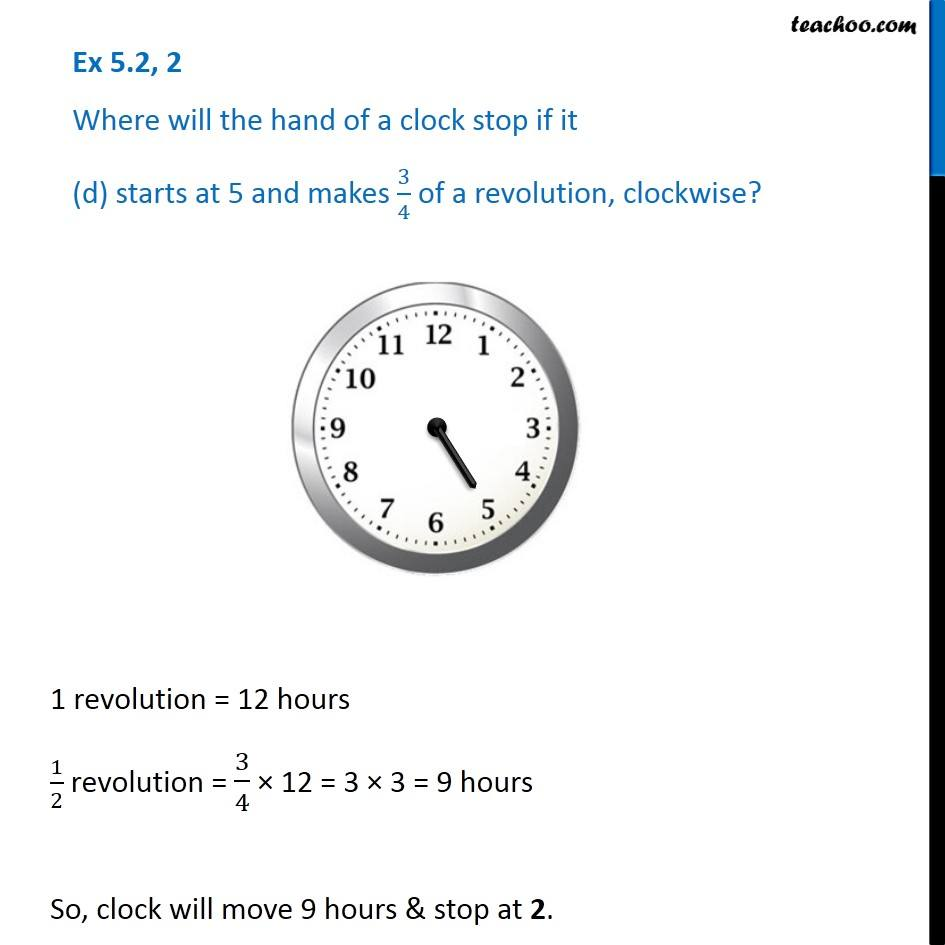Subscribe to our Youtube Channel - https://you.tube/teachoo

1. Chapter 5 Class 6 Understanding Elementary Shapes
2. Serial order wise
3. Ex 5.2

Transcript

Ex 5.2, 2 Where will the hand of a clock stop if it (a) starts at 12 and makes 1/2 of a revolution, clockwise? 1 revolution = 12 hours 1/2 revolution = 12/2 = 6 hours So, clock will move 6 hours & stop at 6. Ex 5.2, 2 Where will the hand of a clock stop if it (b) starts at 2 and makes (1 )/2 of a revolution, clockwise? 1 revolution = 12 hours 1/2 revolution = 6 hours So, clock will move 6 hours & stop at 8. Ex 5.2, 2 Where will the hand of a clock stop if it (c) starts at 5 and makes 1/4 of a revolution, clockwise? 1 revolution = 12 hours 1/2 revolution = 12/4 = 3 hours So, clock will move 3 hours & stop at 8. Ex 5.2, 2 Where will the hand of a clock stop if it (d) starts at 5 and makes 3/4 of a revolution, clockwise? 1 revolution = 12 hours 1/2 revolution = 3/4 × 12 = 3 × 3 = 9 hours So, clock will move 9 hours & stop at 2.

Ex 5.2